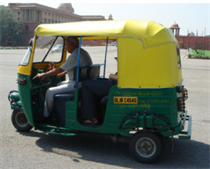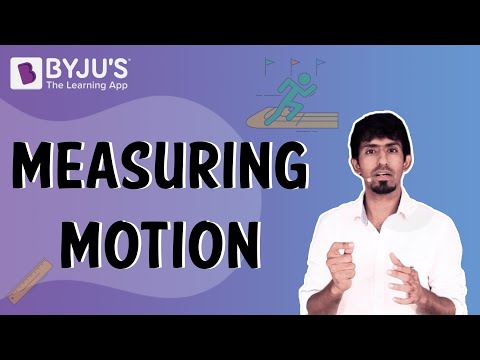Checkout JEE MAINS 2022 Question Paper Analysis : Checkout JEE MAINS 2022 Question Paper Analysis :

# Speed Of Moving Objects

We encounter different events in our day-to-day life where we can witness different types of motion, let’s us take few examples of moving object.Speed of moving objects

## Examples of moving object

An auto-rickshaw can move slow or fast depending upon the traffic, an auto-rickshaw can move faster than a bicycle but an aeroplane can move faster than the auto-rickshaw, how can we decide if one object is moving faster or slower than the other?

On a road we can easily spot the difference in speed of a different moving object by simply looking at them, if we look at the position of vehicles at one instance of time and then again at the same vehicles after 5 seconds we can spot which vehicle moves the faster and which vehicle moves the slower, the distance moved by an object in a given instance of time will help us decide its speed, like for example in a 100 m race, we can say who has the highest speed by looking at who covers 100 m in the shortest time if we have an idea of time and distance we can easily determine the speed.

## Speed

We are familiar with the different moving object and their speed by now, higher speed means that a given distance has been covered in a shorter time or more distance has been covered in the given time, the best way to measure the speed of two objects is to have uniformity in measuring units, say we will take the constant time of 1 hour and measure the distance travelled by the object in that time, we have 3 vehicles,

1. A bicycle can travel a distance of 10 km in 1 hour.
2. An autorickshaw can travel 50 km in 1 hour.
3. An aeroplane can travel 1500 km in 1 hour.

Now, which of the above had greater speed? The answer is c) An aeroplane, because it covered more distance in a given time, we can denote this as the speed of the aeroplane to be 1500 km/hr but that doesn’t mean that the aeroplane travels at a constant speed all the time, the speed may or may not vary during the flight, but on average, the speed at which the aeroplane has travelled is 1500 km/hr we shall use the term speed to determine average speed, and speed can be calculated as,

speed =

$$\begin{array}{l} \frac {distance~travelled}{time~taken}\end{array}$$

In everyday life we hardly find objects moving long distances at a constant speed, objects moving in a straight line at a constant speed are said to have uniform motion, whereas an object that moves in a straight line with varying speed is said to be in Non-uniform motion.

## How to measure motion?## Frequently Asked Questions – FAQs

### What is speed?

Speed can be considered as the rate at which a body covers distance.

### What is the most common formula for calculating the speed of a moving body?

Speed is normally calculated by dividing the distance travelled by the time spent travelling the particular distance.
$$\begin{array}{l}Speed = \frac{Distance}{Time}\end{array}$$

### What is velocity?

Velocity can be defined as the directional speed of a body in movement as an implication if the body’s rate of change in position is perceived from a particular point of reference and as calculated by a specific standard of time. Example: 125km/h to the south.

### What is meant by acceleration?

Acceleration is the rate at which velocity varies with time (both direction and speed).

### What is momentum?

In Newtonian mechanics, momentum is the product of the mass of the moving body and its velocity. It is a vector quantity, having a direction and a magnitude.
Test your Knowledge on Slow and fast motion